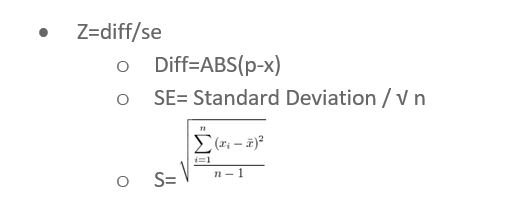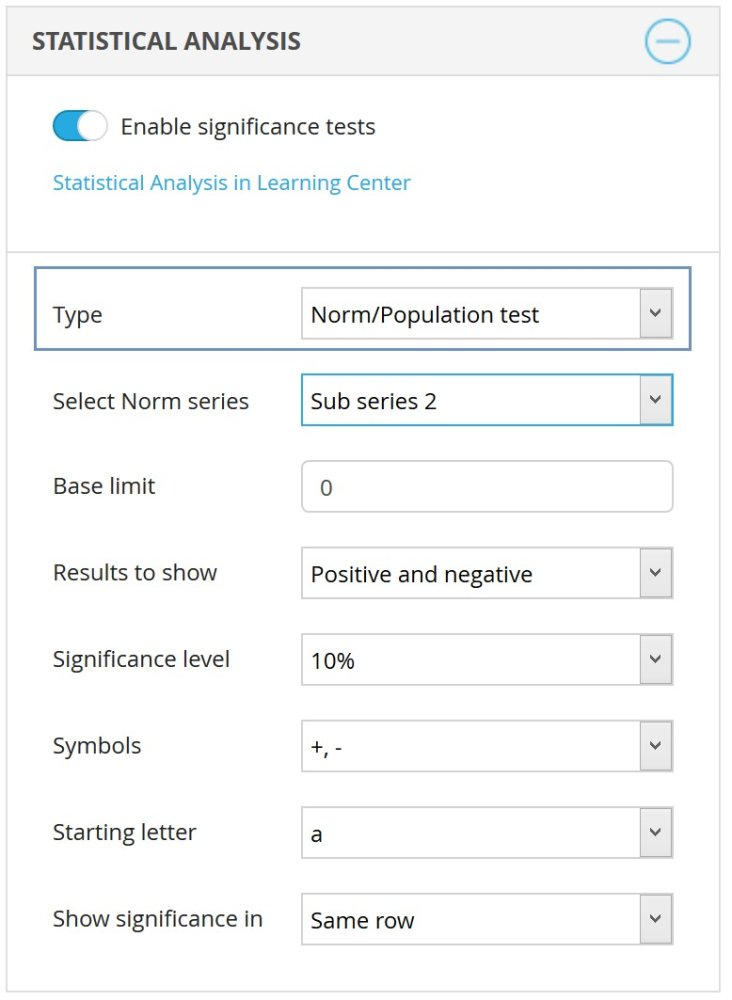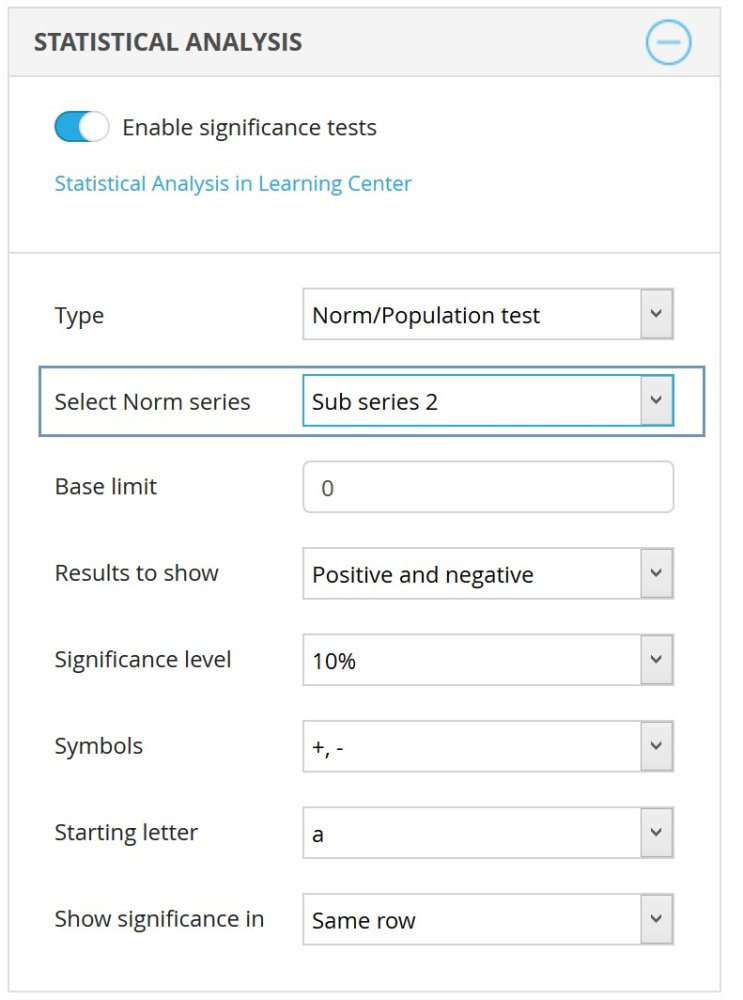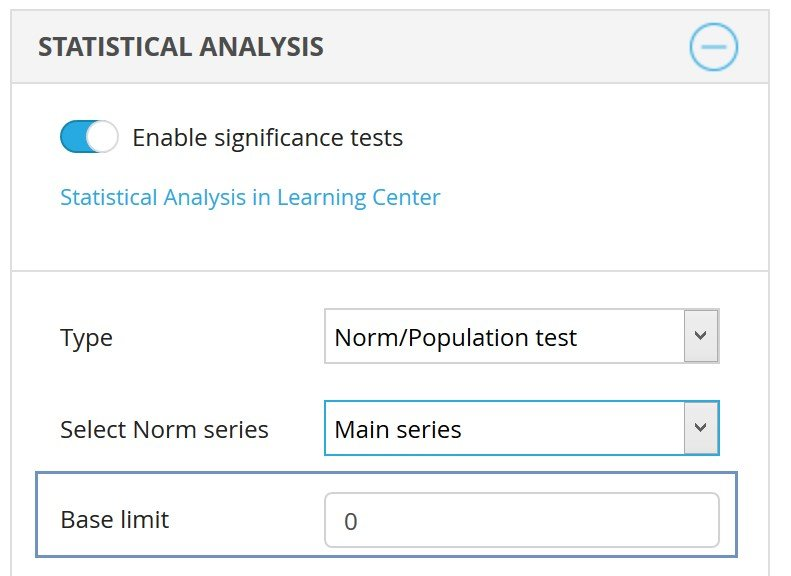The following input values are used in the formula:

p= norm value
x= tested mean value
n= base size* of X
SE = Standard error* of X
S= Standard deviation* of X

FormulaNote: The base size, the standard error, and the standard deviation are based on weighted values if the test value (x) is weighted.

To run a Norm/Population test, the chart/table needs to have at least two series and one of the series needs to use the “Mean – Categorical” calculation or the “Percentage share – categorical” calculation.

Follow these 3 steps to run the Population/Norm test:
1.    Select “Norm/Population test” in Type drop-down list (shown in the image below).

Since the Norm/Population test can only be applied between series, this means it can only be setup in the Main series.2.    Select in which series the Norm values will be found. The values in the other series are then compared to the Norm/Population series cell by cell. This means that cell A1 in the first non-Norm/Population series will be compared to cell A1 in the Norm/Population series, and cell A2 in the first non-Norm/Population series is compared to cell A2 in the Norm/Population series and so on.3.    The rest of the settings are applied just like a regular Two independent sample Z-test

If any base limit is defined (see image below), then it is only applied to the non-Norm/Population series. This is because the Norm/Population values usually only have one respondent in the base size as it is aggregated result calculated outside Dapresy Pro.

The image below highlights the base size settings. This setting is not applied to the series defined as the Norm/Population series.